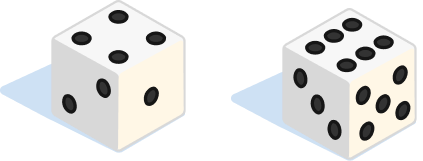### Random Variables & Distributions

Life is unpredictable, and probability is our best mathematical handle on real-world uncertainty.

In this quiz, we'll use simple probabilistic models to introduce random variables, a key concept in quantifying chance outcomes.

# Random Variables

A random variable assigns a numerical value to each outcome of a chance event. Random variables are not the same as the events they quantify.

For example, when a fair coin is flipped exactly twice, the set of all outcomes, or sample space, is $\{ HH, HT, TH, TT \},$ where HH is the event the coin lands heads up on both flips, HT represents a heads followed by a tails, TH a tails followed by heads, and TT is tails on both flips.

HH, HT, TH, TT are not random variables, but the number of heads observed in two flips is. What are some other examples of random variables?

# Random Variables

Select one or more

Let's take a closer look at random variables through an example.

Let $\{ H, T \}$ be the sample space for a single fair coin flip. H and T are not random variables, but if we assign the number 1 to H and the number 0 to T, then we've just created one: $X(H) = 1, \ X(T) = 0.$ $X$ counts the number of heads in a single coin flip.

We don't know what $X$ will be before a flip, but we can compute $P(X=1),$ the probability that we observe $X = 1$ upon flipping the coin. What is $P(X=1) ?$# Random Variables

Let's make things more interesting by flipping the fair coin three times consecutively.

$N,$ the number of tails in three flips, assigns a value to each element in the sample space: $\{\small{ HHH, HHT, HTT, TTT, THH,TTH, HTH, THT} \}.$ For example, $N(HTT) = 2.$ Since fair coin flips are unpredictable, $N$ is a random variable.

Calculate $P(N = 2).$

# Random Variables

Two dice are rolled, and we let $X$ be the sum of the two values that turn up. Find $P( X \geq 10).$ $\\\\$A dice roll with $X = 10$

# Random Variables

Functions create new random variables out of more basic ones.

For example, say Xavier, Yoshi, and Zander all take a multiple-choice test by guessing; their scores are the random variables X, Y, and Z. The average $A = \frac{1}{3} ( X + Y + Z )$ is a function of the original three random variables and is a brand-new random variable on its own.

If the exam has three true/false questions and a score less than $20 \%$ doesn't pass, what is the probability the group average is a failing grade?# Random Variables

So far, all of our random variables have been discrete, meaning their values are countable.

There are many real-world problems best modeled by a continuum of values; we associate to them continuous random variables.

For example, the velocity $V$ of an air molecule inside of a basketball can take on a continuous range of values. We can't know for sure what it is, so $V$ is a continuous random variable.Air molecules moving about with random velocities drawn from a continuum of possibilities

What are some other continuous random variables?

# Random Variables

Select one or more

Let's say a number $x$ is drawn from the interval $[0,1]$ in such a way that if $0 \leq a , b \leq 1,$ then $P( a \leq x \leq b ) = b-a.$ Define the random variable $Y = 2 x + 5.$ What is $P( 6 \leq Y \leq 7) ?$

# Random Variables

Since the number of outcomes is uncountable for a continuous random variable, we need to take a different approach to how we compute its probabilities.

We'll see in the next quiz that dealing with continuous random variables is relatively straightforward if we have a probability distribution.

# Random Variables

×